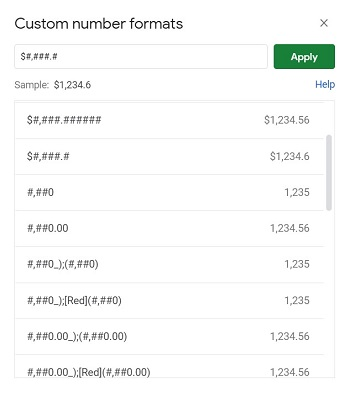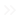Home PC Windows Edge What Does \$ Mean in Google Sheets?

# What Does \$ Mean in Google Sheets?Users of Google Sheets will most likely encounter the dollar symbol the more they use the program. When it appears as part of a number, then it’s can be easily identified as what it is, a symbol for a currency. But what does it mean when \$ shows up in formulas or formatting symbols?

## As an Indicator of Currency

The most obvious meaning of the dollar symbol is that it’s a sign of currency. By default, when a row or column is formatted to display currencies, any number entered will display a dollar sign in front of it.

Currency formatting for Google Sheets follows a few rules. First, decimals only show up to two places, second, any value beyond two decimal places will be rounded, and third, thousand separators are enabled. If someone wishes different formatting rules, they’re going to have to edit a dollar sign on their numbers.## As Part of Number Formatting

When trying to make your own custom number format, you’ll probably encounter the dollar symbol in the format window. Using it means that the current format is meant to apply to currencies, not even necessarily those that use dollars as a symbol.

As mentioned above, currencies are meant to behave a certain way in spreadsheets, and will follow certain rules even when their appearance changes. Seeing the dollar symbol signifies that even though a number will be displayed as formatted, it will retain the behavior of the currency formatting.## As a Static Indicator for Copy-Pasting Formulas

Anyone that uses formulas in Google Sheets may encounter the dollar symbol in terms of duplication. Formulas behave in a certain way when they’re copy-pasted to other cells. This relates to the referencing of cells in certain formulas. For example, if you want to multiply the values of two cells A1 and B1, you write the formula =A1*B1. If you enter this formula in C1 for example, then copy-paste it into cell C2, you’ll get the formula =A2*B2.

Google Sheets automatically assumes that you want to create successive rows of the same formula using different cells. This happens even if you copy multiple formulas at once, each formula referencing a cell at least one value above the first. For the given example, if you copy-pasted the first two formulas =A1*B1 and A2*B2 and pasted them onto cell C3, it will automatically change the formulas into =A3*B3 and =A4*B4.

Similar formatting will be shown when the formula is copy-pasted horizontally. =A1*B1 pasted onto cell D1 will return the formula =B1*C1, and so on.This is all well and good if you actually want this behavior in your copy-pasted formulas, but what if you want some of the variables to change, but some to stay the same? For example, what if you want column C to display cells B1 to B5 multiplied with only A1, or cells D1 to F1 to reference only B1? It would be fine to do so manually, but if you’re dealing with a large spreadsheet this would be inconvenient. It also defeats the point of copy-pasting in the first place. The answer would be the dollar symbol.

When used in formulas, dollar symbols indicate to Google Sheets that the next number or letter to the right of it should remain static. Even when copy pasted, any value without the symbol will increment, and those with it will not. As an example, the formula =\$A1*B\$1 copy pasted to cell D2 will not increment A in A1, or 1 in B1. The resulting formula would be =\$A2*C\$1. As long as there is a \$ before it, that number or letter will not change when pasted into another cell. All other values will increment in the series.This is a handy tool for duplicating large numbers of formulas while setting rules on how they should behave. If you can dictate what exactly changes in a copy pasted formula you eliminate the need to manually adjust them afterwards.

## A Variety of Meanings

People that utilize Google Sheets may be surprised at the variety of meanings that the simple dollar symbol can hold depending on its use. Having the knowledge of how \$ behaves in Google Sheets allows savvy users to take advantage of its capabilities.

Do you know of other meanings for \$ in Google Sheets? Share your thoughts in the comments section below.

#### How to Change Your Text in Snapchat

Read Next